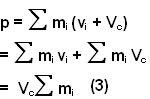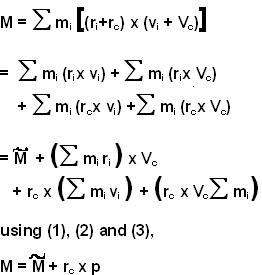## Monday, June 30, 2008

### Irodov Problem 1.197

Let there be n particles in the system and let their masses be m1,m2,...,mn. Let their position vectors as seen from the center of inertia of the system be r1,r2,...,rn and their velocity vectors as seen from the center of inertia be v1,v2,...,vn. From the very definition of moment of inertia we have,Suppose that the velocity vector of the center of inertia of the system of particles with respect to frame K is given by Vc. Then the velocities of the individual particles in the system as seen from the frame K will be the vectors v1+Vc,v2+Vc,...,vn+Vc. The momentum of the system of particles as seen from frame K will then be,The position vectors of the particles as seen from frame K will be r1+rc,r2+rc,...,rn+rc. So, the angular momentum of the system of particles as seen from K will be,